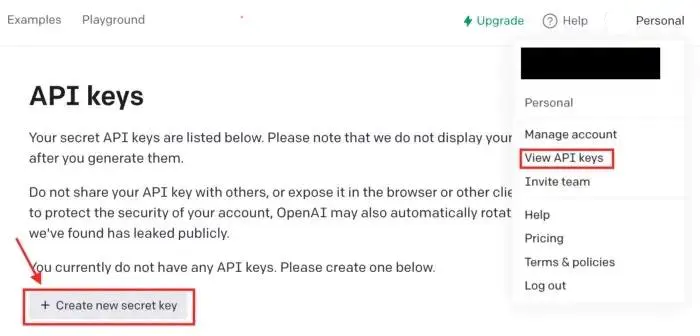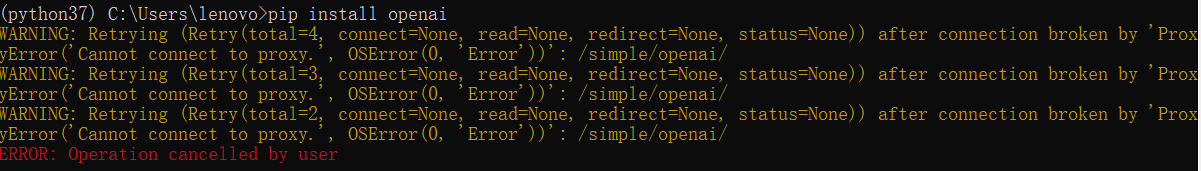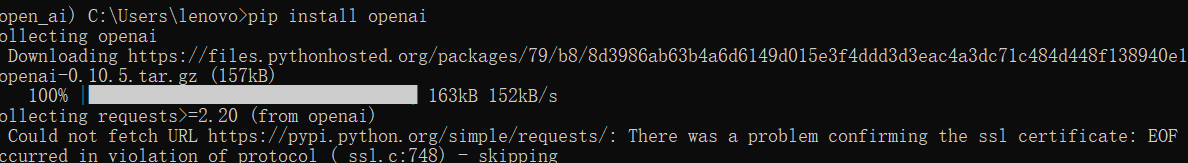# 程序员最近都爱上了这个网站  程序员们快来瞅瞅吧！  it98k网:it98k.com

出租广告位,需要合作请联系站长

+关注

# 生成 API 密钥# 使用 Python接入 OpenAI API

``pip install openai``## 1.文本生成

``import os import openaiopenai.api_key = os.getenv("OPENAI_API_KEY") prompt =  """Decide whether a Mike's sentiment is positive, neutral, or negative. Mike: I don't like homework!Sentiment:""" response = openai.Completion.create( model="text-davinci-003", prompt=prompt, max_tokens=100, temperature=0  )  print(response)``

``{  "choices": [    {      "finish_reason": "stop",      "index": 0,      "logprobs": null,      "text": "Negative"    }  ],...}``

model ：要使用的模型的 ID（在这里你可以看到所有可用的模型）

Prompt：生成结果的触发指令

max_token：完成时生成的最大token数量（这里可以看到OpenAI使用的tokenizer）

temperature：要使用的采样策略。 接近 1 的值会给模型带来更多风险/创造力，而接近 0 的值会生成明确定义的答案。

## 2. 代码生成

Codex 模型系列是经过自然语言和数十亿行代码训练的 GPT-3 系列的后代。 借助 Codex，我们可以将注释转化为代码、重写代码以提高效率等等。

``import osimport openai openai.api_key = os.getenv("OPENAI_API_KEY") response = openai.Completion.create(  model="code-davinci-002",  prompt="\"\"\"\nCreate an array of weather temperatures for Shanghai\n\"\"\"",  temperature=0,  max_tokens=256,  top_p=1,  frequency_penalty=0,  presence_penalty=0) print(response)``

``{  "choices": [    {      "finish_reason": "stop",      "index": 0,      "logprobs": null,      "text": "\n\nimport numpy as np\n\ndef create_temperatures(n):\n    \"\"\"\n    Create an array of weather temperatures for Shanghai\n    \"\"\"\n    temperatures = np.random.uniform(low=14.0, high=20.0, size=n)\n    return temperatures"    }  ],...  }}``

``import numpy as np def create_temperatures(n):    temperatures = np.random.uniform(low=14.0, high=20.0, size=n)    return temperatures``

## 3. 图像生成

``import openai response = openai.Image.create(  prompt="A fluffy white cat with blue eyes sitting in a basket of flowers, looking up adorably at the camera",  n=1,  size="1024x1024")image_url = response['data']['url']print(image_url)``4 0

pdf(new) 更多>